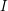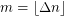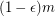# Hardness of Approximation

## Refuting random 3SAT-instances on $O(n)$ clauses (weak form) ★★★

Author(s): Feige

Conjecture   For every rationaland every rational, there is no polynomial-time algorithm for the following problem.

Given is a 3SAT (3CNF) formulaonvariables, for some, andclauses drawn uniformly at random from the set of formulas onvariables. Return with probability at least 0.5 (over the instances) thatis typical without returning typical for any instance with at leastsimultaneously satisfiable clauses.

Keywords: NP; randomness in TCS; satisfiability